# Sperner property

(diff) ← Older revision | Latest revision (diff) | Newer revision → (diff)

Letbe a finite partially ordered set (abbreviated: poset) which possesses a rank function, i.e. a function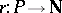such thatfor some minimal elementofand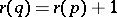whenevercovers, i.e.and there is no element betweenand. Letbe itsth level and let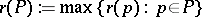be the rank of.

An anti-chain or Sperner family inis a subset of pairwise incomparable elements of. Obviously, each level is an anti-chain. The width (Dilworth number or Sperner number) ofis the maximum sizeof an anti-chain of. The posetis said to have the Sperner property if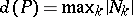. E. Sperner proved in 1928 the Sperner property for Boolean lattices (cf. also Sperner theorem).

More generally, a-family,, is a subset ofcontaining no chain ofelements in, andhas the strong Sperner property if for eachthe largest size of a-family inequals the largest size of a union oflevels. There exist several classes of posets having the strong Sperner property:

LYM posets, i.e. posetssatisfying the LYM inequality (cf. also Sperner theorem)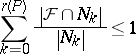for every anti-chain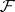inor, equivalently,for all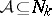,, where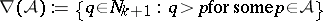. This equivalent property is called the normalized matching property of.

Symmetric chain orders, i.e. ranked posetswhich can be decomposed into chains of the form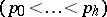where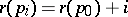,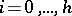, and.

Peck posets, i.e. ranked posetssuch that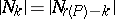for alland there is a linear operator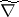on the vector space having the basiswith the following properties:with some numbers,

the subspace generated by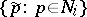is mapped via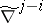to a subspace of dimension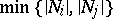for all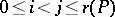. Ifandare posets from one class, then also the direct product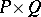(ordered componentwise) belongs to that class, where in the case of LYM posets an additional condition must be supposed: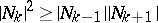for all(so-called logarithmic concavity). Moreover, quotient theorems have been proved for LYM posets with weight functions and Peck posets.

Every LYM poset with the symmetry and unimodality property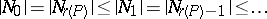is a symmetric chain order and every symmetric chain order is a Peck poset.

Standard examples of posets belonging to all these three classes are the lattice of subsets of a finite set, ordered by inclusion (the Boolean lattice), the lattice of divisors of a natural number, ordered by divisibility, the lattice of all subspaces of an-dimensional vector space over a finite field, ordered by inclusion. The poset of faces of an-dimensional cube, ordered by inclusion, belongs only to the class of LYM posets. The lattice of partitions of a finite set, ordered by refinement, even does not have the Sperner property ifis sufficiently large.

Details can be found in [a1].

How to Cite This Entry:
Sperner property. Encyclopedia of Mathematics. URL: http://encyclopediaofmath.org/index.php?title=Sperner_property&oldid=18446
This article was adapted from an original article by K. Engel (originator), which appeared in Encyclopedia of Mathematics - ISBN 1402006098. See original article